# (Tutorial) Gamma Correction: A Story of Linearity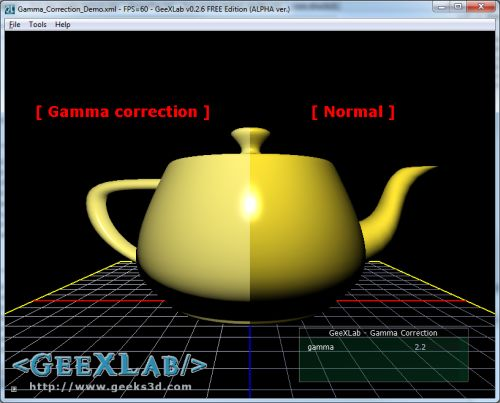Before writing this tutorial, I didn’t think that gamma correction could affect the final image so much. The left part of the header image shows a gamma corrected rendering while the right part shows a non-gamma corrected rendering. The left part is the real rendering.

## What is gamma correction and why is it important?

Gamma correction is the operation that aims to cancel the non-linearity property of a display device (CRT or LCD). There are other devices that display an image but in this article I focuse only on monitors.

The following graph shows the non-linear response of a monitor: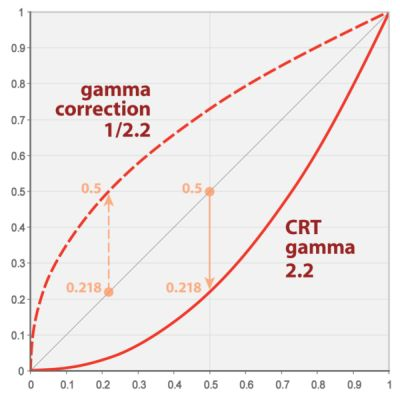(source)

A monitor has a non linear response. That means if we sum two colors, the color of the pixel displayed will not be the sum of both colors but will have a lesser value.

Here is a simple example. Let’s consider we have an Add() function that compute the sum of two channels (here the red channel). Channels values are in the range [0.0 ; 1.0]

```red = Add(r1, r2)
...
```

With a linear device, we’ll get: red = 0.391. With a monitor, we’ll get red = 0.126. Why?

Because the response of a monitor can be approximated by a power function like this:

C_out = C_in2.2

2.2 is the gamma factor of the monitor. Actually, the gamma factor is in the range of 2.0 to 2.4 depending on the monitor. Gamma correction must cancel the effect of gamma factor. This is done wit the following equation:

C_corrected= C_out1.0/2.2

Ok let’s plug some numbers in these equations:

• 0.3912.2 = 0.126
• 0.1261.0/2.2 = 0.39

• 0.3911.0/2.2 = 0.652
• 0.652 – 0.391 = 0.261
• 0.391 – 0.126 = 0.265

As you can see, if we raise the monitor response value (0.126) to the power of 1/2.2, we get the right value (0.39): we have gamma-corrected the monitor output!

Enough with the theory, let’s see how we can do this gamma correction in practice for a real time 3D application.

## How to achieve gamma correction?

There are two ways to do gamma correction:

• Using the renderer. The renderer (the graphics card or GPU) is a linear device. Modern renderers have the support of gamma correction via sRGB textures and framebuffer formats. See the following OpenGL extensions for more details: GL_ARB_framebuffer_sRGB and GL_EXT_texture_sRGB. With these extensions you can get gamma corrected values for free but gamma correction factor is set to 2.2. You can’t change it.
• Using a software gamma correction. The gamma correction is applied to the final scene buffer thanks to a pixel shader and you can set the gamma correction you want.

I coded a small GeeXLab demo that shows the impact of gamma correction (or the impact of the non-linear response of the monitor as you want). You can download the demo at the end of the article.

The demo is quite simple. I render a simple scene (a yellow teapot + the reference grid) into a frame buffer. The final scene image is got by applying a gamma correction shader on the scene buffer.

In this demo, the gamma correction is done with a post processing filter. Here is the GLSL shader that concretely performs the gamma correction:

```[Vertex_Shader]
void main(void)
{
gl_Position = ftransform();
gl_TexCoord = gl_MultiTexCoord0;
}

uniform sampler2D sceneBuffer;
uniform float gamma;
void main(void)
{
vec2 uv = gl_TexCoord.xy;
vec3 color = texture2D(sceneBuffer, uv).rgb;
if (uv.x<0.50)
gl_FragColor.rgb = pow(color, vec3(1.0 / gamma));
else
gl_FragColor.rgb = color;
gl_FragColor.a = 1.0;
}
```

The gamma factor is an uniform variable so you can change it (with the tweak bar in the GeeXlab demo) and see the impact of different values.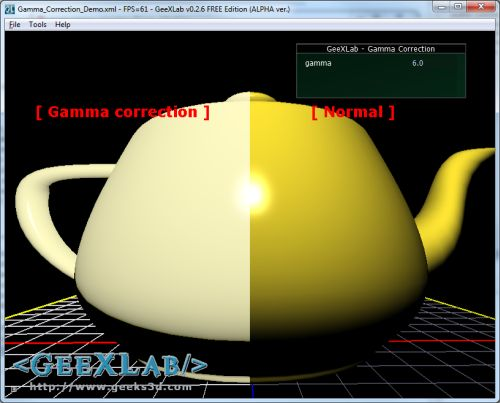gamma correction of 6.0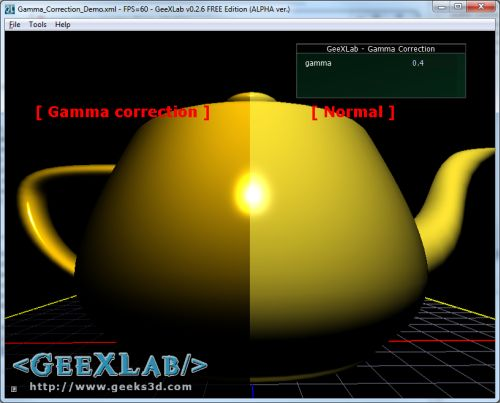gamma correction of 0.4

Now, if we want to be more precise, we have to apply gamma-correction to each channel (red, green and blue) separately (just drag n drop the source file Gamma_Correction_Channel_Demo_SRC.xml in GeeXLab):

```[Vertex_Shader]
void main(void)
{
gl_Position = ftransform();
gl_TexCoord = gl_MultiTexCoord0;
}

uniform sampler2D sceneBuffer;
uniform vec3 gammaRGB;
void main(void)
{
vec2 uv = gl_TexCoord.xy;
vec3 color = texture2D(sceneBuffer, uv).rgb;
if (uv.x<0.50)
gl_FragColor.rgb = pow(color, 1.0 / gammaRGB);
else
gl_FragColor.rgb = color;
gl_FragColor.a = 1.0;
}
```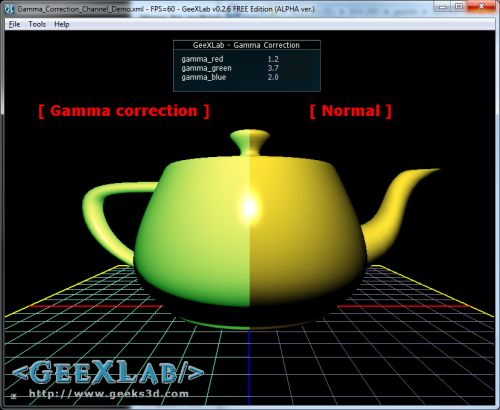Here is the gamma correction with GL_ARB_framebuffer_sRGB (demo Gamma_Correction_sRGB_Demo.xml):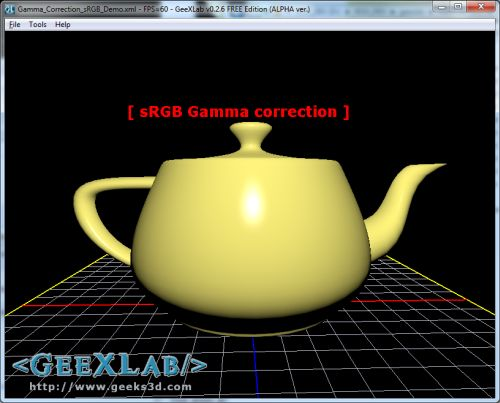Gamma correction using GL_ARB_framebuffer_sRGB

In OpenGL, using GL_ARB_framebuffer_sRGB is really simple: once your FBO is bound, just enable the sRGB space with

```glEnable(GL_FRAMEBUFFER_SRGB);
```

With GeeXLab, you can use sRGB space with the gamma_correction attribute of a render_texture node:

```<render_texture name="sceneBuffer"
type="FRAMEBUFFER" gamma_correction="TRUE" >
<attach_texture_color pixel_format="RGBA_FLOAT"/>
<attach_render_buffer type="DEPTH" pixel_format="DEPTH24"/>
</render_texture>
```

Hey, but I'm not a coder, is there another way to apply the gamma correction? Yes, in the control panel of display drivers. For example, in NVIDIA control panel you'll find gamma correction in the "Adjust desktop color settings" section. By default gamma is set to 1.0 (non correction):Now if we set gamma to 2.2 we get the same rendering than in the left part of the demo.

Some video games also offer gamma correction setting.

As you can see, there is a notable difference between gamma corrected and non-gamma corrected images. Gamma correction is simple solution to improve visual quality in real time 3D apps.

The following image shows an important difference in the self-shadow boundary between gamma and non-gamma corrected images: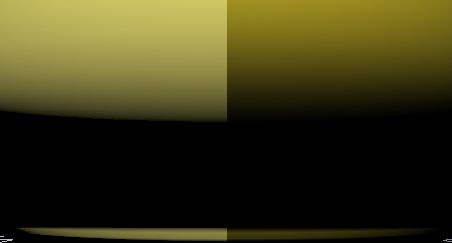Just unzip the demo somewhere and load the Gamma_Correction_Demo_SRC.xml or Gamma_Correction_Channel_Demo.xml file in GeeXLab 0.2.6 or newer. You can also drop the demos in GeeXLab to execute them.

## 11 thoughts on “(Tutorial) Gamma Correction: A Story of Linearity”

1.jarik

I’ve played with vidoe-card gamma correction settings. Changing it from 1.0 to 2.2 caused image over-burned (too bright). Is it possible that LCD monitors do correction automaticly ?

2.FMoreira

XBOX 360 and D3D >= 10 hardware does this automatically 🙂
That’s why some times ago the first users of games that used D3D10 as the rendering API complained about brighter images 😛

3.Matumbo

If you want your gamma corrected result to keep the same yellow color that appears in the non gamma corrected side, you can apply the pow(2.2) to your input color. That way you’ll see the exact same color, but with the sharp fallof and the beautiful spec highlight.

About DirectX10, I think it also applies the inverse gamma after the blending stage, so that the blending is also gamma correct.

4.Dave

Gamma Correction Demo doesn’t work here (ATI mobility 5650). Errors in GammaCorrectionShader:
ERROR: 0:7: error(#202) No matching overloaded function found pow
ERROR: 0:7: error(#160) Cannot convert from ‘const float’ to ‘3-component vector of float’

Dave

5.Stefan

Same issue with ATI Mobility 5470.
Channel demo works fine though.

6.Maurice

In these examples you are computing a linear image, while your display device expects a non-linear image. That’s why the image look too bright. Gamma correction is used such that a pixel intensity of 128 looks approximately twice as bright as 64. The latter is one of the assumptions in the Phong shading model you use in your examples, yet it no longer holds in the images you display.

As I understood things, gamma correction often refers to performing arithmetic with colors (like averaging, or adding as you mentioned) in linear space. That means you transform the input colors to linear space (^2.2), average them, and then convert the output back to non-linear space (^(1.0/2.2)). That’s because with averaging you should indeed take the actual amount of light emitted by the display device into account, not the perceived brightness.

7.waldyk

Well, I’ve always been increasing gamma values for my CTX CRT monitor, because otherwise it was not only too dark (i remember that pain when playing shooter\action game, the dark scene – and u don’t see anything and then u get killed), but also realism suffered as well – trees, landscapes appeared artificially saturated, skin tone appeared sometomes too red, sometimes yellowish, overally the image looked like underexposed photo. Mostly affected by this issue are whose Trinitron tubes.
Now, owing a notebook with lcd display, I must say what u absolutely don’t need any upping of gamma levels, moreover i actually lowered my gamma setts for it, because otherwise it’s not just too bright, but, given the fact it’s TN, colors in the bright areas are literally saying undistinguishable! So if u got LCD with TN matrix in it, u should try lowering gamma – it will visually improve picture AND if u own a hi quality PVA/MVA or IPS one then u should no worry at all – in most cases gamma and colors in them are precise enough. Also i must tell that none of the monitor types (LCD vs CRT, TN vs IPS vs *VA) do any gamma correction – all differences are just because of differencies in technologies of picture formation on the screen.
P.S. Sorry for mistakes/misspelling (East Europe! :D)

8.Lightness1024

9.sfsdf